Create a new printableProbability
Math Worksheets

Sample - Click above to make a new math worksheet (PDF).
 Name _____________________________Date ___________________
Probability
Write whether each event is certain, likely, unlikely, or impossible.
1.
 The following coins are put in a bag: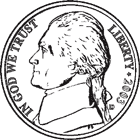Describe the probability of picking a quarter.2.
 A glass jar contains a total of 31 marbles. The jar has green and red marbles. There are 5 red marbles. Describe the probability of picking a blue marble.3.
 A glass jar contains a total of 36 marbles. The jar has red and blue marbles. There are 28 red marbles. Describe the probability of picking a yellow marble.4 * This is a pre-made sheet.Use the link at the top of the page for a printable page.

Write the probability.
1.   If one letter is chosen at random from the word assassinate, what is the probability that the letter chosen is the letter "t"?
 ____ out of   11
2.   If one letter is chosen at random from the word superintendent, what is the probability that the letter chosen is the letter "d"?
 ____ out of   14
3.   A number cube has 6 sides. The sides have the numbers 9, 9, 5, 8, 3, and 6. If the cube is thrown once, what is the probability of rolling an odd number?
 4   out of ____
4.   A bag contains 18 red marbles, 18 purple marbles, 10 yellow marbles, 5 green marbles, and 15 blue marbles. What is the probability of pulling out a yellow marble?
 10   out of ____

Write the probability.
1.   If one letter is chosen at random from the word crushing, what is the probability that the letter chosen is the letter "n"?
 ____ out of ____
2.   A number cube has 6 sides. The sides are numbered 1 to 6. If the cube is thrown once, what is the probability of rolling the number 1?
 ____ out of ____
3.   A bag contains 12 red marbles, 9 yellow marbles, 17 blue marbles, 19 green marbles, and 11 purple marbles. What is the probability of pulling out a red or a blue marble?
 ____ out of ____
4.   If one letter is chosen at random from the word independence, what is the probability that the letter chosen is the letter "e"?
 ____ out of ____

 Key #2
 aKey #2
Write the probability of spinning each letter.
1.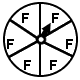The letter F.
 ____ out of   6
2.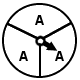The letter A.
 3   out of ____
3.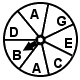The letter D.
 1   out of ____
4.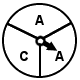The letter A.
 ____ out of   3

Write whether each event is certain, likely, unlikely, or impossible.
1.
 The following coins are put in a bag: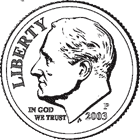Describe the probability of picking a nickel.2.
 The following coins are put in a bag: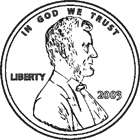Describe the probability of picking a penny.Write the probability of spinning each letter.
1.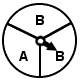The letter A.
 ____ out of ____
2.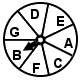The letter D.
 ____ out of ____
3.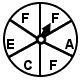The letter F.
 ____ out of ____
4.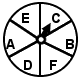The letter F.
 ____ out of ____

Write the probability.
1.   If one letter is chosen at random from the word endeavored, what is the probability that the letter chosen is the letter "r"?
 ____ out of ____
2.   A number cube has 6 sides. The sides are numbered 1 to 6. If the cube is thrown once, what is the probability of rolling an odd number?
 ____ out of ____
3.   A bag contains 13 purple marbles, 18 yellow marbles, 13 blue marbles, 12 red marbles, and 19 green marbles. What is the probability of pulling out a purple or a blue marble?
 ____ out of ____
4.   If one letter is chosen at random from the word pleat, what is the probability that the letter chosen is the letter "p"?
 ____ out of ____